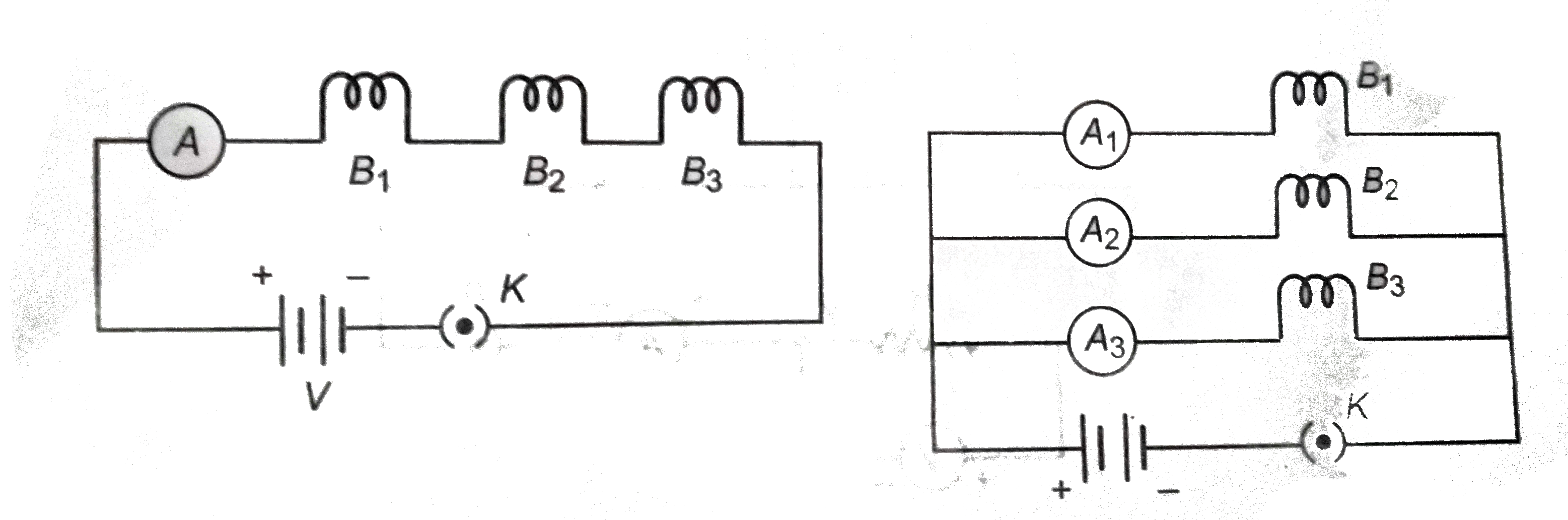Doubtnut App - Get 50 Lakh+ video solutions better than GoogleInstall Now

# Three incandecent bulbs of 100W each are connected in series in an electric circuit. In another circuit another set of three bulbs of the same wattage are connected in parallel to the same source. (a) Will the bulbs in the two circuits glow with the same brightness ? Justify your answer. (b) Now let one bulb in both the circuits get fused. Will the rest of the bulbs continue to glow in each circuit ? Give reason.

Text Solution
Verified by Experts

## (a) Let us assume that the resistance of each bulb be R. The circuit diagram in two cases may be drawn as given belowEquivalent resistance in series combination R3=R+R+R=3R, voltage = V Let current through each bulb in series combination be I1. By Ohm's law, V=I1×3R⇒I1=V3R So, power consumption of each bulb in series combination. P1=I21(3R)=(V3R)2×3R=V29R2×3R =V23R=V23R ....(i) For parallel circuit, (1) The resistance of each bulb = R Voltage across each bulb = V [∵same voltage in parallel combination] ∴ Power consumption of each bulb in parallel combination. P2=V2R ....(ii) ∵ From Eqs. (i) and (ii). we have P2P1=V2/RV2/3R V2R×3RV2=3⇒P2=3P1 Therefore, each bulb in parallel combination glows 3 times brighter to that of each bulb in series combination. (b) When one bulb fused in both the circuit, then in series combination, circuit gets broken and current stops flowing and remaining bulb don't glow. Whereas in parallel combination, same voltage continue to act on the remaining voltage and hence, other bulbs continue to glow with same brightness.